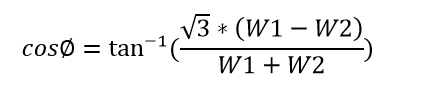# V Curve & Inverted V Curve of Synchronous Motor Why?

### What is V Curve and inverted v curve?

The graphical representation of armature current Ia vs field current If is called V-curve since the final view looks like English letter V. At the same time the power factor vs field current is called inverted V-curve of a synchronous motor.

Just you connect, two three-phase wattmeters and an ammeter to the stator winding of the synchronous motor. Also, connect another ammeter to the field input supply. Continue this experiment, with different field current as well as various load conditions such as load, half load and full load.

Here stator side connected ammeter gives you armature current Ia and field-side connected ammeter gives you field current If,

#### V curve Ia Vs If:

Take If reading at x-axis and Ia reading at Y-axis. Connect all the reading points, we get a V-shaped curve for a synchronous motor is shown in Figure 1.1.

At no-load condition, the real power supplied to the machine is zero since Ia=0, at unity power factor. When you increase the field current above that point, the line current increases with respect to applied field current, (Here the reactive current flows from the motor to source). in such case, the synchronous motor can be used as a synchronous capacitor.

At load condition, the motor draws a small amount of real current at unity power factor. After plotting the graph, the same V shape is still maintained.

#### Inverted V-curve:

Now take the reading of the power factor in the y-axis and field current in the x-axis and plot a graph. Look at the shape of the graph, it seems like inverted V.